# # 图结构

TIP

## # 特点

• 一组顶点：通常用 V （Vertex）表示顶点的集合
• 一组边：通常用 E （Edge）表示边的集合
1. 边是顶点和顶点之间的连线
2. 边可以是有向的，也可以是无向的。比如 A----B 表示无向，A ---> B 表示有向

## # 常用术语

• 顶点：表示图中的一个节点
• 边：表示顶点和顶点给之间的连线
• 相邻顶点：由一条边连接在一起的两个顶点
• 度：一个顶点的度是相邻顶点的数量
• 路径：
1. 简单路径：简单路径要求不包含重复的顶点
2. 回路：第一个顶点和最后一个顶点相同的路径称为回路
• 无向图：图中的所有边都是没有方向的
• 有向图：图中的所有边都是有方向的
• 无权图：无权图中的边没有任何权重意义
• 带权图：带权图中的边有一定的权重含义

## # 表示方法

### # 邻接矩阵

• 一种表示图的常用方式
• 可以使用二维数组来表示邻接矩阵
• 邻接矩阵让每个节点和一个整数相关联，该整数作为数组的下标值
• 使用一个二维数组来表示顶点之间的连接
• 邻接矩阵的问题
1. 如果图是一个稀疏图，那么邻接矩阵中将存在大量的 0，会造成存储空间的浪费
2. 而且即使只有一个边, 我们也必须遍历一行来找出这个边, 也浪费很多时间• 二维数组中的 0 表示没有连线，1 表示有连线
• 如：A[ 0 ][ 3 ] = 1，表示 A 和 C 之间有连接
• 邻接矩阵的对角线上的值都为 0，表示 A - A ，B - B，等自回路都没有连接（自己与自己之间没有连接）
• 若为无向图，则邻接矩阵应为对角线上元素全为 0 的对称矩阵
• 上图很多 0 就会出现空间浪费

### # 邻接表

• 一种表示图的常用方式
• 邻接表由图中每个顶点以及和顶点相邻的顶点列表组成
• 这个列表可用多种方式存储，比如：数组/链表/字典（哈希表）等都可以
• 邻接表的问题
1. 邻接表计算出度是比较简单的(出度: 指向别人的数量, 入度: 指向自己的数量)
2. 邻接表如果需要计算有向图的入度, 就必须构造一个“逆邻接表”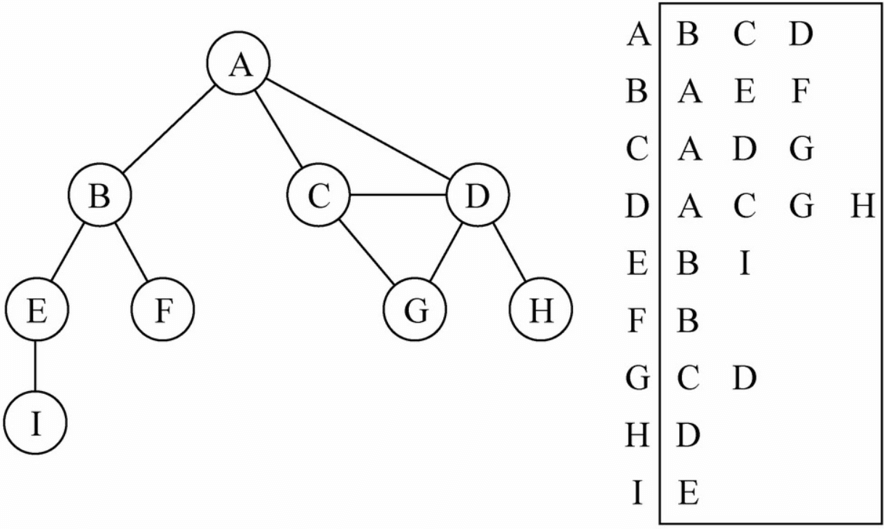• 图中可清楚看到 A 与 B、C、D 相邻
• 假如要表示这些与 A 顶点相邻的顶点（边），可以通过将它们作为 A 的值（value）存入到对应的数组/链表/字典中
• 之后，通过键（key）A 可以十分方便地取出对应的数据

## # 代码封装

### # 创建图类

``````// 封装图类
function Graph() {
// 属性：顶点(数组)/边(字典)
this.vertexes = [] // 存储顶点
this.adjList = new Dictionay() // 存储边
}
``````

### # 添加顶点和边

• 创建一个数组对象 vertexes 存储图的顶点
• 创建一个字典对象 edges 存储图的边，其中 key 为顶点，value 为存储 key 顶点相邻顶点的数组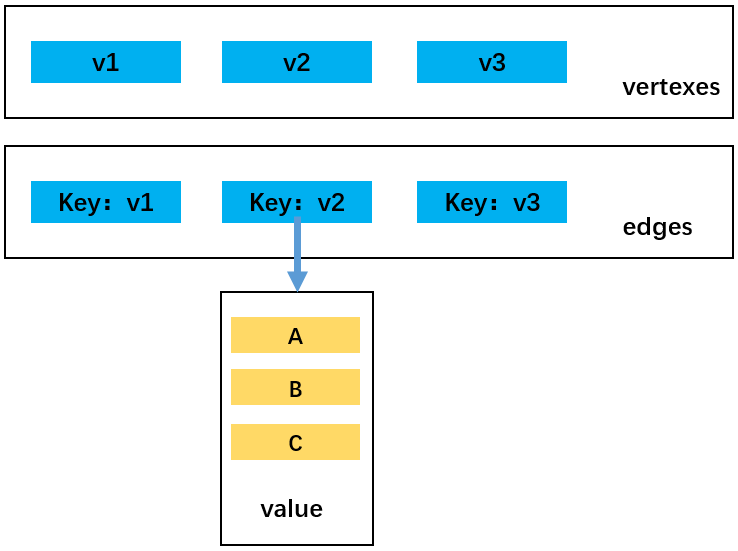``````// 1. 添加顶点
this.vertexes.push(v)
// 将边添加到字典中，新增的顶点作为键，对应的值为一个存储边的空数组
this.edges.set(v, [])
}

// 2. 添加边：传入两个顶点为它们添加边
Graph.prototype.addEdge = function (v1, v2) {
// 取出字典对象edges中存储边的数组，并添加关联顶点
this.edges.get(v1).push(v2)
// 表示的是无向表，故要添加互相指向的两条边
this.edges.get(v2).push(v1)
}
``````

### # 转换为字符串输出

``````// 3. 实现toString方法:转换为字符串输出
Graph.prototype.toString = function () {
// 3.1 定义字符串，保存最终结果
var resultString = ""

// 3.2 遍历所有的顶点以及顶点对应的边
for (var i = 0; i < this.vertexes.length; i++) {
// 遍历顶点
resultString += this.vertexes[i] + "-->"
// 取出边数组
var vEdges = this.edges.get(this.vertexes[i])
for (var j = 0; j < vEdges.length; j++) {
//遍历字典中每个顶点对应的数组
resultString += vEdges[j] + "  "
}
resultString += "\n"
}
// 3.3 返回结果
return resultString
}
``````

``````// 测试代码
var graph = new Graph()

// 1. 添加顶点
var myVertexes = ["A", "B", "C", "D", "E", "F", "G", "H", "I"]
for (var i = 0; i < myVertexes.length; i++) {
}

// 2. 添加边

// 3. 测试toString方法
console.log(graph.toString())
``````

``````A-->B  C  D
B-->A  E  F
C-->A  D  G
D-->A  C  G  H
E-->B  I
F-->B
G-->C  D
H-->D
I-->E
``````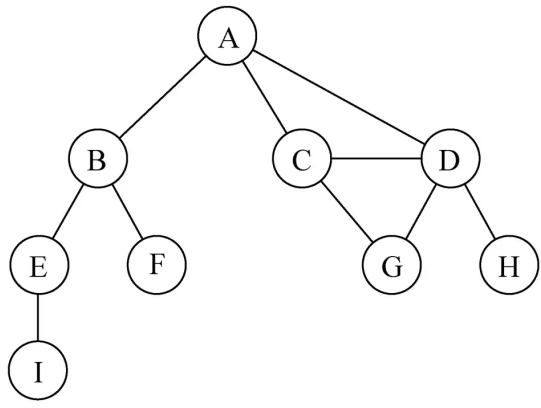### # 遍历说明

• 广度优先搜索（Breadth - First Search，简称 BFS）
• 深度优先搜索（Depth - First Search，简称 DFS）
• 两种遍历算法都需要指定第一个被访问的顶点

• BFS: 基于队列, 入队列的顶点先被探索
• DFS: 基于栈, 通过将顶点存入栈中, 顶点是沿着路径被探索的, 存在新的相邻顶点就去访问

• 白色：表示该顶点还没有被访问过
• 灰色：表示该顶点被访问过，但并未完全被访问过
• 黑色：表示该顶点被访问过，且都被访问过

``````// 4. 初始化状态颜色: 全部初始化为白色
Graph.prototype.initializeColor = function () {
var colors = []
for (var i = 0; i < this.vertexes.length; i++) {
colors[this.vertexes[i]] = "white"
}
return colors
}
``````

### # 广度优先搜索

• 先宽后深的访问顶点：从指定的第一个顶点开始遍历图, 先访问其所有的相邻点, 然后一层一层遍历下去，直到遍历结束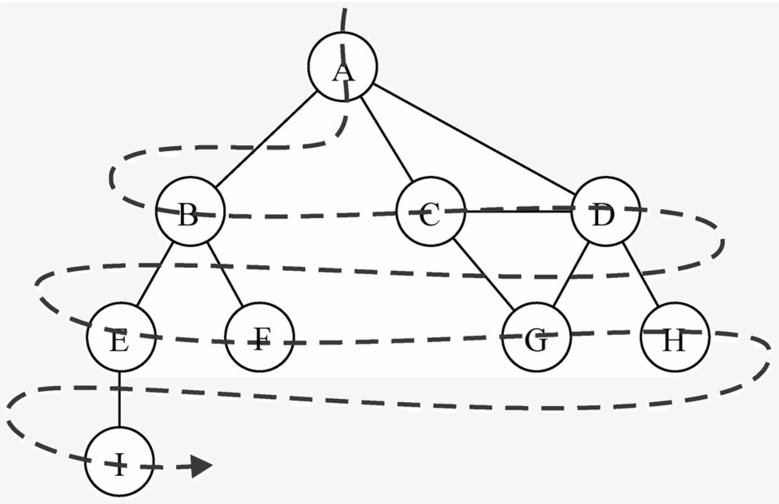• 基于队列实现广度优先搜索算法
• 首先，创建一个队列 Q
• 其次，将 v 标注为被发现的(灰色), 并将 v 将入队列 Q
• 再次，如果 Q 非空, 执行下面的步骤:
1. 将 v 从 Q 中取出队列
2. 将 v 标注为被发现的灰色
3. 将 v 所有的未被访问过的邻接点(白色), 加入到队列中
4. 将 v 标志为黑色

``````// 5. 实现广度优先搜索(BFS)：传入指定的第一个顶点、处理结果的函数
Graph.prototype.bfs = function (initV, handler) {
// 5.1 初始化颜色
var colors = this.initializeColor()

// 5.2 创建队列
var queue = new Queue()

// 5.3 将顶点放入队列中
queue.enqueue(initV)

// 5.4 循环从队列中取出元素
while (!queue.isEmpty()) {
// 5.4.1 从队列中取出一个顶点
var v = queue.dequeue()

// 5.4.2 获取和顶点相邻的另外顶点
var vList = this.edges.get(v)

// 5.4.3 将v的颜色设置为灰色
colors[v] = "gray"

// 5.4.4 遍历v所有相邻的顶点，并加入到队列中
for (var i = 0; i < vList.length; i++) {
var e = vList[i]
// 判断相邻顶点是否被探测过，被探测过则不加入队列中；并且加入队列后变为灰色，表示被探测过
if (colors[e] == "white") {
colors[e] = "gray"
queue.enqueue(e)
}
}

// 5.4.4 访问顶点
handler(v)

// 5.4.5 将顶点v设置为黑色。此时黑色顶点v位于队列最前面，进入下一次while循环时会被取出
colors[v] = "black"
}
}
``````

• 下为指定的第一个顶点为 A 时的遍历过程：
1. 如 a 图所示，将在字典 edges 中取出的与 A 相邻的且未被访问过的白色顶点 B、C、D 放入队列 que 中并变为灰色，随后将 A 变为黑色并移出队列
2. 如 b 图所示，将在字典 edges 中取出的与 B 相邻的且未被访问过的白色顶点 E、F 放入队列 que 中并变为灰色，随后将 B 变为黑色并移出队列
3. 如 c 图所示，将在字典 edges 中取出的与 C 相邻的且未被访问过的白色顶点 G（A，D 也相邻不过已变为灰色，所以不加入队列）放入队列 que 中并变为灰色，随后将 C 变为黑色并移出队列
4. 如 d 图所示，将在字典 edges 中取出的与 D 相邻的且未被访问过的白色顶点 H 放入队列 que 中并变为灰色，随后将 D 变为黑色并移出队列
• 如此循环直到队列中元素为 0，即所有顶点都变黑并移出队列后才停止，此时图中顶点已被全部遍历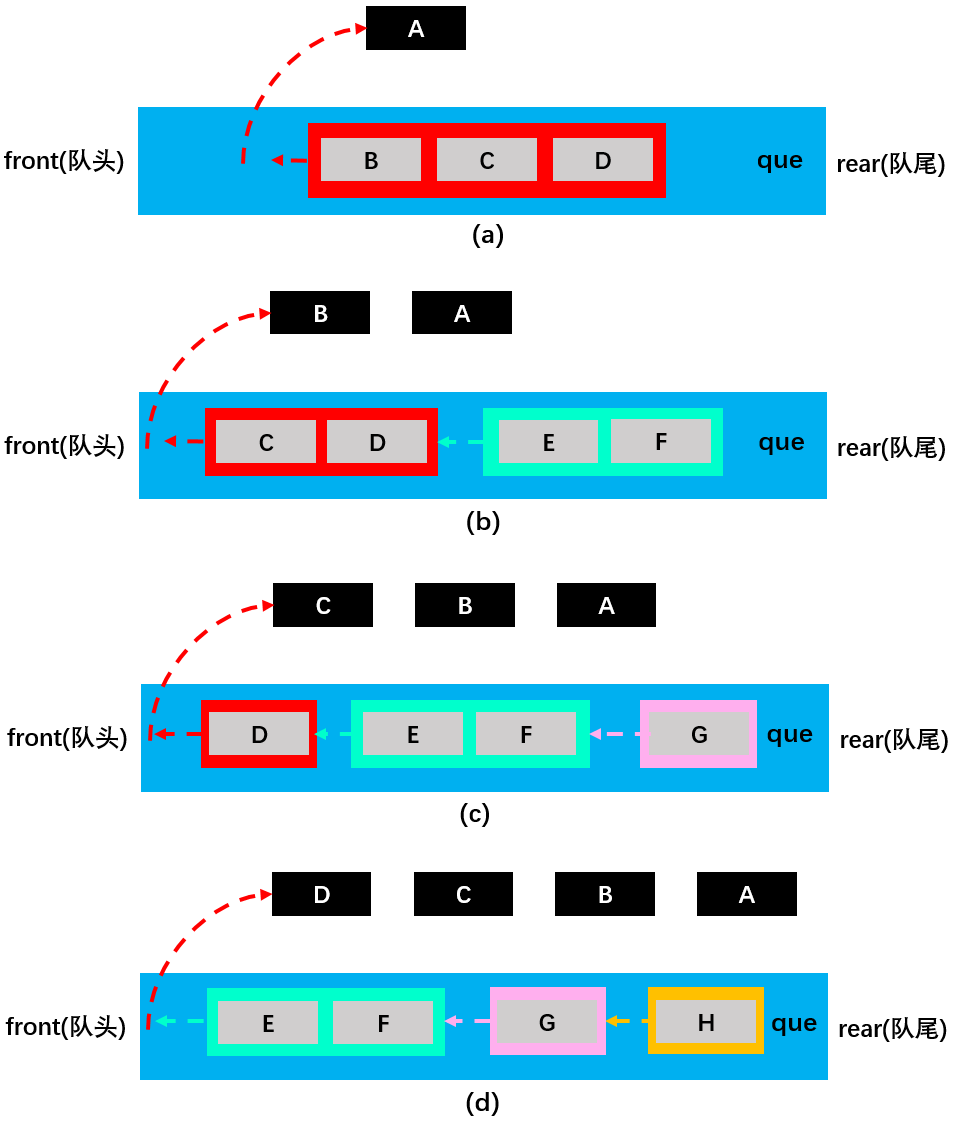``````// 4. 测试bfs：调用广度优先算法
var result = ""
graph.bfs(graph.vertexes, function (v) {
result += v + " -> "
})
console.log(result) // A -> B -> C -> D -> E -> F -> G -> H -> I ->
``````

### # 深度优先搜索

• 先深后宽的访问顶点：从指定的第一个顶点开始遍历图, 先沿着一条路径遍历直到该路径的最后一个顶点都被访问过为止，然后沿原来路径回退并探索下一条路径，直到遍历完全部节点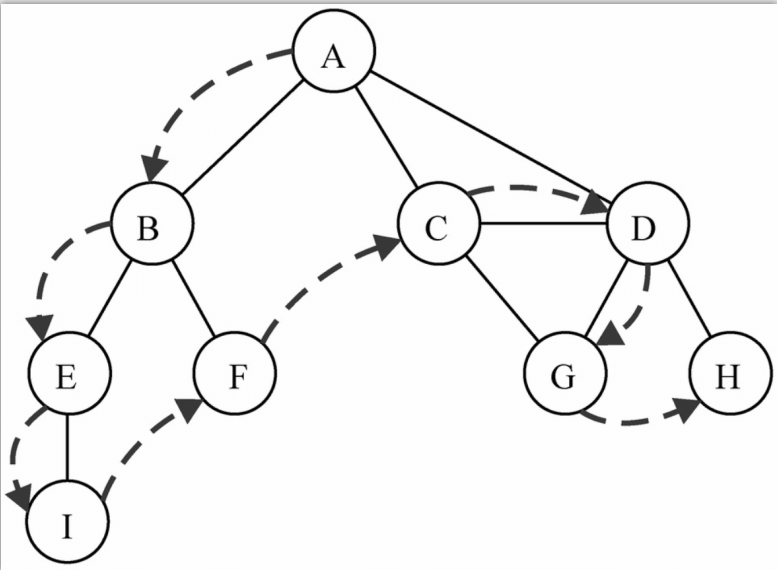• 可以使用栈结构来实现深度优先搜索算法
• 深度优先搜索算法的遍历顺序与二叉搜索树中的先序遍历较为相似，同样可以使用递归来实现（递归的本质就是函数栈的调用）
• 基于递归实现深度优先搜索算法：定义 dfs 方法用于调用递归方法 dfsVisit，定义 dfsVisit 方法用于递归访问图中的各个顶点
• 首先，在 dfs 方法中：
1. 调用 initializeColor 方法将所有顶点初始化为白色
2. 调用 dfsVisit 方法遍历图的顶点
• 其次，在 dfsVisit 方法中：
1. 将传入的指定节点 v 标注为灰色
2. 处理顶点 V
3. 访问 V 的相邻顶点
4. 将顶点 v 标注为黑色

``````// 6. 实现深度优先搜索(DFS)：传入指定的第一个顶点、处理结果的函数
Graph.prototype.dfs = function (initV, handler) {
// 6.1 初始化颜色
var colors = this.initializeColor()

// 6.2 从某个顶点开始依次递归访问
this.dfsVisit(initV, colors, handler)
}
// 递归访问方法，传入三个参数分别表示：指定的第一个顶点、颜色、处理函数
Graph.prototype.dfsVisit = function (v, colors, handler) {
// a. 将颜色设置为灰色
colors[v] = "gray"

// b. 处理v顶点
handler(v)

// c. 访问v相连的顶点
var vList = this.edges.get(v)
for (var i = 0; i < vList.length; i++) {
var e = vList[i]
// 判断相邻顶点是否为白色，若为白色，递归调用函数继续访问
if (colors[e] == "white") {
this.dfsVisit(e, colors, handler)
}
}

// d. 将v设置为黑色
colors[v] = "black"
}
``````

• 下为代码中的第 c 步操作：访问指定顶点的相邻顶点：
1. 以指定顶点 A 为例，先从储存顶点及其对应相邻顶点的字典对象 edges 中取出由顶点 A 的相邻顶点组成的数组[B, C, D]
2. 第一步：A 顶点变为灰色，随后进入第一个 for 循环，遍历 A 白色的相邻顶点：B、C、D；在该 for 循环的第 1 次循环中（执行 B），B 顶点满足：colors == "white"，触发递归，重新调用该方法
3. 第二步：B 顶点变为灰色，随后进入第二个 for 循环，遍历 B 白色的相邻顶点：E、F；在该 for 循环的第 1 次循环中（执行 E），E 顶点满足：colors == "white"，触发递归，重新调用该方法
4. 第三步：E 顶点变为灰色，随后进入第三个 for 循环，遍历 E 白色的相邻顶点：I；在该 for 循环的第 1 次循环中（执行 I），I 顶点满足：colors == "white"，触发递归，重新调用该方法
5. 第四步：I 顶点变为灰色，随后进入第四个 for 循环，由于顶点 I 的相邻顶点 E 不满足：colors == "white"，停止递归调用
6. 递归结束后一路向上返回，首先回到第三个 for 循环中继续执行其中的第 2、3...次循环，每次循环的执行过程与上面的同理，直到递归再次结束后，再返回到第二个 for 循环中继续执行其中的第 2、3...次循环....以此类推直到将图的所有顶点访问完为止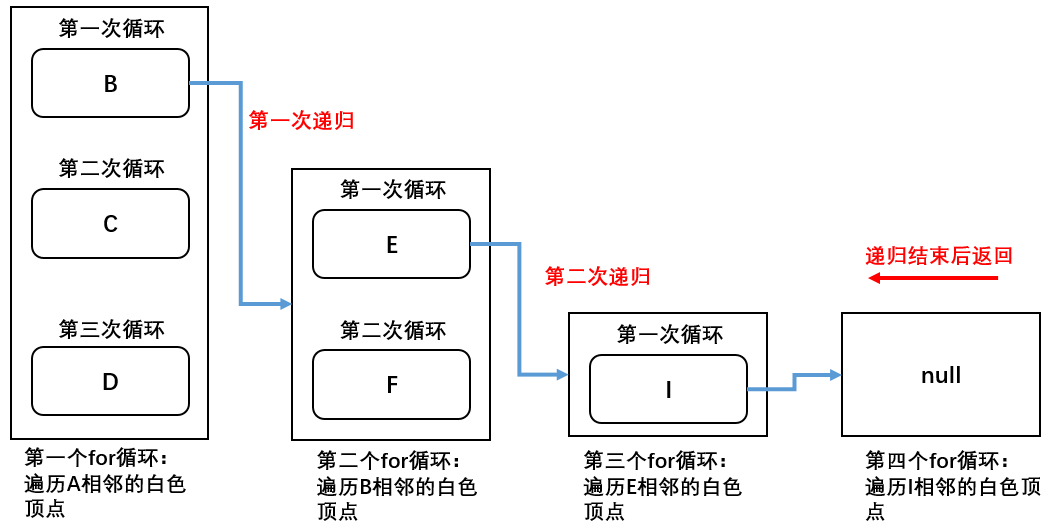• 发现 表示访问了该顶点，状态变为灰色
• 探索 表示既访问了该顶点，也访问了该顶点的全部相邻顶点，状态变为黑色
• 由于在顶点变为灰色后就调用了处理函数 handler，所以 handler 方法的输出顺序为发现顶点的顺序即：A、B、E、I、F、C、D、G、H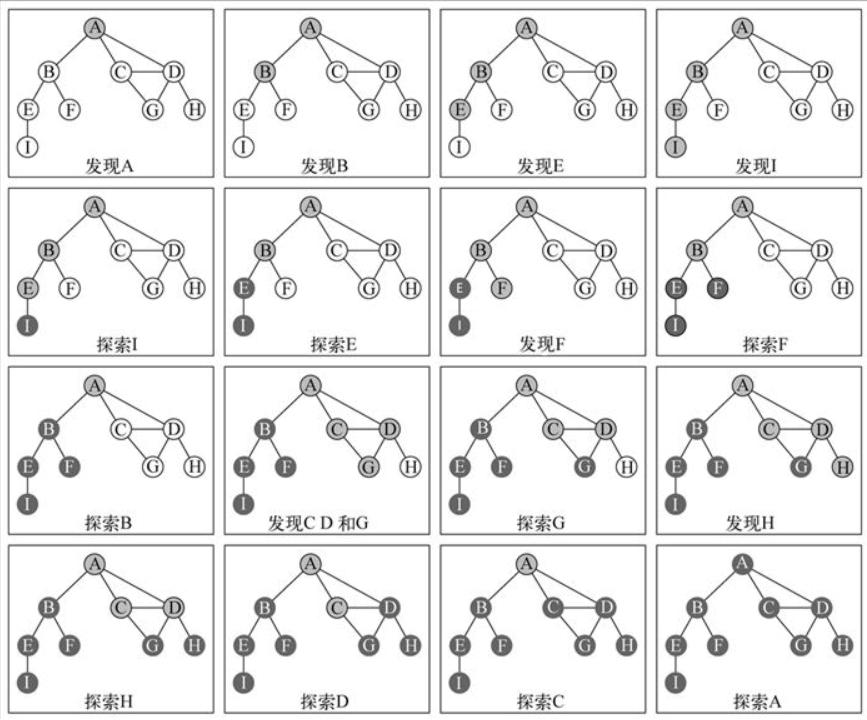``````// 5. 测试dfs：调用深度优先算法
result = ""
graph.dfs(graph.vertexes, function (v) {
result += v + " -> "
})
console.log(result) // A -> B -> E -> I -> F -> C -> D -> G -> H ->
``````

### # 完整代码

``````// 封装图类
function Graph() {
// 属性：顶点(数组)/边(字典)
this.vertexes = [] // 存储顶点
this.edges = new Dictionay() // 存储边

/* 方法 */
// 1. 添加顶点
this.vertexes.push(v)
// 将边添加到字典中，新增的顶点作为键，对应的值为一个存储边的空数组
this.edges.set(v, [])
}

// 2. 添加边：传入两个顶点为它们添加边
Graph.prototype.addEdge = function (v1, v2) {
// 取出字典对象edges中存储边的数组，并添加关联顶点
this.edges.get(v1).push(v2)
// 表示的是无向表，故要添加互相指向的两条边
this.edges.get(v2).push(v1)
}

// 3. 实现toString方法:转换为字符串输出
Graph.prototype.toString = function () {
// 3.1 定义字符串，保存最终结果
var resultString = ""

// 3.2 遍历所有的顶点以及顶点对应的边
for (var i = 0; i < this.vertexes.length; i++) {
// 遍历顶点
resultString += this.vertexes[i] + "-->"
// 取出边数组
var vEdges = this.edges.get(this.vertexes[i])
for (var j = 0; j < vEdges.length; j++) {
//遍历字典中每个顶点对应的数组
resultString += vEdges[j] + "  "
}
resultString += "\n"
}
// 3.3 返回结果
return resultString
}

// 4. 初始化状态颜色: 全部初始化为白色
Graph.prototype.initializeColor = function () {
var colors = []
for (var i = 0; i < this.vertexes.length; i++) {
colors[this.vertexes[i]] = "white"
}
return colors
}

// 5. 实现广度优先搜索(BFS)：传入指定的第一个顶点、处理结果的函数
Graph.prototype.bfs = function (initV, handler) {
// 5.1 初始化颜色
var colors = this.initializeColor()

// 5.2 创建队列
var queue = new Queue()

// 5.3 将顶点放入队列中
queue.enqueue(initV)

// 5.4 循环从队列中取出元素
while (!queue.isEmpty()) {
// 5.4.1 从队列中取出一个顶点
var v = queue.dequeue()

// 5.4.2 获取和顶点相邻的另外顶点
var vList = this.edges.get(v)

// 5.4.3 将v的颜色设置为灰色
colors[v] = "gray"

// 5.4.4 遍历v所有相邻的顶点，并加入到队列中
for (var i = 0; i < vList.length; i++) {
var e = vList[i]
// 判断相邻顶点是否被探测过，被探测过则不加入队列中；并且加入队列后变为灰色，表示被探测过
if (colors[e] == "white") {
colors[e] = "gray"
queue.enqueue(e)
}
}

// 5.4.4 访问顶点
handler(v)

// 5.4.5 将顶点v设置为黑色。此时黑色顶点v位于队列最前面，进入下一次while循环时会被取出
colors[v] = "black"
}
}

// 6. 实现深度优先搜索(DFS)：传入指定的第一个顶点、处理结果的函数
Graph.prototype.dfs = function (initV, handler) {
// 6.1 初始化颜色
var colors = this.initializeColor()

// 6.2 从某个顶点开始依次递归访问
this.dfsVisit(initV, colors, handler)
}
// 递归访问方法，传入三个参数分别表示：指定的第一个顶点、颜色、处理函数
Graph.prototype.dfsVisit = function (v, colors, handler) {
// a. 将颜色设置为灰色
colors[v] = "gray"

// b. 处理v顶点
handler(v)

// c. 访问v相连的顶点
var vList = this.edges.get(v)
for (var i = 0; i < vList.length; i++) {
var e = vList[i]
// 判断相邻顶点是否为白色，若为白色，递归调用函数继续访问
if (colors[e] == "white") {
this.dfsVisit(e, colors, handler)
}
}

// d. 将v设置为黑色
colors[v] = "black"
}
}

// 测试代码
var graph = new Graph()

// 1. 添加顶点
var myVertexes = ["A", "B", "C", "D", "E", "F", "G", "H", "I"]
for (var i = 0; i < myVertexes.length; i++) {
}

// 2. 添加边

// 3. 测试toString方法
console.log(graph.toString())

// 4. 测试bfs：调用广度优先算法
var result = ""
graph.bfs(graph.vertexes, function (v) {
result += v + " -> "
})
console.log(result) // A -> B -> C -> D -> E -> F -> G -> H -> I ->

// 5. 测试dfs：调用深度优先算法
result = ""
graph.dfs(graph.vertexes, function (v) {
result += v + " -> "
})
console.log(result) // A -> B -> E -> I -> F -> C -> D -> G -> H ->
``````